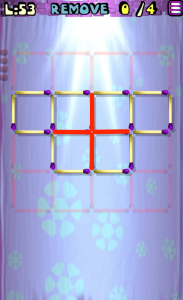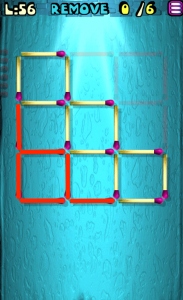# Move the Matches Puzzles 41-60 Walkthrough

Walkthrough for all the solutions and answers to the game Move the Matches Puzzles by K-Lab. Get the app on the Google Play Store to play along if you do not already have it.

### Level 41Instructions: Remove 2 Matches to correct the equation

Solution: 1+3=4

### Level 42Instructions: Move 2 matches to correct the equations

Solution: 8+8=16

### Level 43Instructions: Remove 3 matches to get 4 triangles

### Level 44Instructions: Move 1 match to get 4 squares, all equal

### Level 45Instructions: Add 1 match to correct the equation

Solution: 7×4=28

### Level 46Instructions: Move 2 matches to correct the equation

Solution: 0+2=2

### Level 47Instructions: Add 3 matches to get 4 triangles

### Level 48Instructions: Add 2 matches to get 3 triangles

### Level 49Instructions: Move 2 matches to correct the equation

Solution: 2+2=4

### Level 50Instructions: Move 4 matches to get 3 squares

### Level 51Instructions: Move 1 match to correct the equation

Solution: 4+5=9

### Level 52Instructions: Move 2 matches to correct the equation

Solution: 8/8=1

### Level 53Instructions: Remove 4 matches to get 3 squares

### Level 54Instructions: Add 1 match to correct the equation

Solution: 9+9=18

### Level 55Instructions: Move 2 matches to get 2 squares

### Level 56Instructions: Remove 6 matches to get 3 squares, all equal

### Level 57Instructions: Move 2 matches to get 3 triangles

### Level 58Instructions: Add 1 match to correct the equation

Solution: 7/1=7

### Level 59Instructions: Move 2 matches to correct the equation

Solution: 8/2=4

### Level 60Instructions: Move 4 matches to get 2 squares